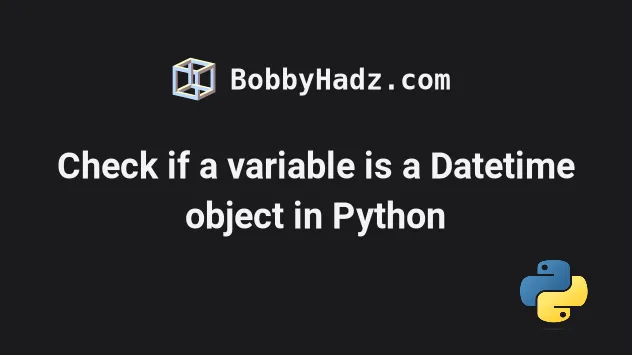# Check if a variable is a datetime object in PythonWed Apr 20 20222 min read## Check if a variable is a datetime object in Python#

Use the `isinstance` built-in function to check if a variable is a datetime object in Python, e.g. `if isinstance(today, datetime):`. The `isinstance` function returns `True` if the passed in object is an instance or a subclass of the passed in class.

main.py
```Copied!```from datetime import datetime

today = datetime.today()

print(today)

if isinstance(today, datetime):
# 👇️ this runs
print('variable is datetime object')
else:
print('variable is NOT datetime object')
``````

The isinstance function returns `True` if the passed in object is an instance or a subclass of the passed in class.

We imported the `datetime` class from the datetime module.

The `datetime` class is a subclass of the `date` class, so make sure you pass the correct second argument to `isinstance`.

If you create a `date` object and check if it's an instance of `datetime`, you would get `False` back.

main.py
```Copied!```from datetime import datetime, date

d = date(2022, 9, 24)

if isinstance(d, datetime):
print('variable is datetime object')
else:
# 👇️ this runs
print('variable is NOT datetime object')
``````

The `else` block runs because `date` objects are not an instance of `datetime`, they are an instance of the `date` class.

main.py
```Copied!```from datetime import  date

d = date(2022, 9, 24)

# 👇️ pass date as second arg to isinstance
if isinstance(d, date):
# 👇️ this runs
print('variable is datetime object')
else:
print('variable is NOT datetime object')
``````

You can also use the `is` operator to check if an object is a `datetime` object.

main.py
```Copied!```from datetime import datetime, date

d = date(2022, 9, 24)
print(type(d) is date)  # 👉️ True

today = datetime.today()
print(type(today) is datetime)  # 👉️ True
``````

The is and is not operators test for an object's identity: `x is y` is true only if `x` and `y` are the same object.

An object's identity is determined using the id function.

`x is not y` returns the inverse truth value.

The type class returns the type of an object.

main.py
```Copied!```from datetime import datetime, date

d = date(2022, 9, 24)
print(type(d))  # 👉️ <class 'datetime.date'>

today = datetime.today()
print(type(today))  # 👉️ <class 'datetime.datetime'>
``````

Most commonly the return value is the same as accessing the `__class__` attribute on the object.

Use the search field on my Home Page to filter through my more than 1,000 articles.